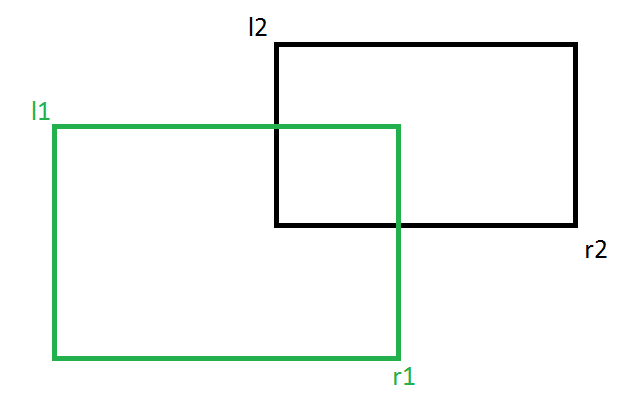Open In App
Related Articles

# Find if two rectangles overlap

Given two rectangles, find if the given two rectangles overlap or not.
Note that a rectangle can be represented by two coordinates, top left and bottom right. So mainly we are given following four coordinates.
l1: Top Left coordinate of first rectangle.
r1: Bottom Right coordinate of first rectangle.
l2: Top Left coordinate of second rectangle.
r2: Bottom Right coordinate of second rectangle.We need to write a function bool doOverlap(l1, r1, l2, r2) that returns true if the two given rectangles overlap.

Recommended Practice

Note : It may be assumed that the rectangles are parallel to the coordinate axis.
One solution is to one by one pick all points of one rectangle and see if the point lies inside the other rectangle or not. This can be done using the algorithm discussed here
Following is a simpler approach. Two rectangles do not overlap if one of the following conditions is true.
1) One rectangle is above top edge of other rectangle.
2) One rectangle is on left side of left edge of other rectangle.
We need to check above cases to find out if given rectangles overlap or not. Following is the implementation of the above approach.

## C++

 `// CPP program for the above approach``#include ` `struct` `Point {``    ``int` `x, y;``};` `// Returns true if two rectangles (l1, r1) and (l2, r2)``// overlap``bool` `doOverlap(Point l1, Point r1, Point l2, Point r2)``{``    ``// if rectangle has area 0, no overlap``    ``if` `(l1.x == r1.x || l1.y == r1.y || r2.x == l2.x || l2.y == r2.y)``        ``return` `false``;``  ` `    ``// If one rectangle is on left side of other``    ``if` `(l1.x > r2.x || l2.x > r1.x)``        ``return` `false``;` `    ``// If one rectangle is above other``    ``if` `(r1.y > l2.y || r2.y > l1.y)``        ``return` `false``;` `    ``return` `true``;``}` `/* Driver program to test above function */``int` `main()``{``    ``Point l1 = { 0, 10 }, r1 = { 10, 0 };``    ``Point l2 = { 5, 5 }, r2 = { 15, 0 };``    ``if` `(doOverlap(l1, r1, l2, r2))``        ``printf``(``"Rectangles Overlap"``);``    ``else``        ``printf``(``"Rectangles Don't Overlap"``);``    ``return` `0;``}`

## Java

 `// Java program to check if rectangles overlap  ``class` `GFG {` `   ``static` `class` `Point {` `        ``int` `x, y;``    ``}` `// Returns true if two rectangles (l1, r1) and (l2, r2) overlap`` ``static`  `boolean` `doOverlap(Point l1, Point r1, Point l2, Point r2) {``        ``// if rectangle has area 0, no overlap``        ``if` `(l1.x == r1.x || l1.y == r1.y || r2.x == l2.x || l2.y == r2.y)``            ``return` `false``;``    ` `           ``// If one rectangle is on left side of other``        ``if` `(l1.x > r2.x || l2.x > r1.x) {``            ``return` `false``;``        ``}` `        ``// If one rectangle is above other``        ``if` `(r1.y > l2.y || r2.y > l1.y) {``            ``return` `false``;``        ``}` `        ``return` `true``;``    ``}` `    ``/* Driver program to test above function */``    ``public` `static` `void` `main(String[] args) {``        ``Point l1 = ``new` `Point(),r1 = ``new` `Point(),``                ``l2 = ``new` `Point(),r2 = ``new` `Point();``        ``l1.x=``0``;l1.y=``10``; r1.x=``10``;r1.y=``0``;``         ``l2.x=``5``;l2.y=``5``; r2.x=``15``;r2.y=``0``;` `        ``if` `(doOverlap(l1, r1, l2, r2)) {``            ``System.out.println(``"Rectangles Overlap"``);``        ``} ``else` `{``            ``System.out.println(``"Rectangles Don't Overlap"``);``        ``}``    ``}``}``//this code contributed by PrinciRaj1992`

## Python3

 `# Python program to check if rectangles overlap``class` `Point:``    ``def` `__init__(``self``, x, y):``        ``self``.x ``=` `x``        ``self``.y ``=` `y` `# Returns true if two rectangles(l1, r1)``# and (l2, r2) overlap``def` `do_overlap(l1, r1, l2, r2):``    ` `    ``# if rectangle has area 0, no overlap``    ``if` `l1.x ``=``=` `r1.x ``or` `l1.y ``=``=` `r1.y ``or` `r2.x ``=``=` `l2.x ``or` `l2.y ``=``=` `r2.y:``        ``return` `False``    ` `    ``# If one rectangle is on left side of other``    ``if` `l1.x > r2.x ``or` `l2.x > r1.x:``        ``return` `False` `    ``# If one rectangle is above other``    ``if` `r1.y > l2.y ``or` `r2.y > l1.y:``        ``return` `False` `    ``return` `True` `# Driver Code``if` `__name__ ``=``=` `"__main__"``:``    ``l1 ``=` `Point(``0``, ``10``)``    ``r1 ``=` `Point(``10``, ``0``)``    ``l2 ``=` `Point(``5``, ``5``)``    ``r2 ``=` `Point(``15``, ``0``)` `    ``if``(do_overlap(l1, r1, l2, r2)):``        ``print``(``"Rectangles Overlap"``)``    ``else``:``        ``print``(``"Rectangles Don't Overlap"``)` `# This code is contributed by Vivek Kumar Singh`

## C#

 `// C# program to check if rectangles overlap``using` `System;``    ` `class` `GFG``{``    ``class` `Point``    ``{``        ``public` `int` `x, y;``    ``}` `    ``// Returns true if two rectangles (l1, r1)``    ``// and (l2, r2) overlap``    ``static` `bool` `doOverlap(Point l1, Point r1,``                          ``Point l2, Point r2)``    ``{``        ``// if rectangle has area 0, no overlap``        ``if` `(l1.x == r1.x || l1.y == r1.y || r2.x == l2.x || l2.y == r2.y)``        ``{``            ``return` `false``;``        ``}``      ` `        ``// If one rectangle is on left side of other``        ``if` `(l1.x > r2.x || l2.x > r1.x)``        ``{``            ``return` `false``;``        ``}` `        ``// If one rectangle is above other``        ``if` `(r1.y > l2.y || r2.y > l1.y)``        ``{``            ``return` `false``;``        ``}``        ``return` `true``;``    ``}` `    ``// Driver Code``    ``public` `static` `void` `Main()``    ``{``        ``Point l1 = ``new` `Point(), r1 = ``new` `Point(),``                ``l2 = ``new` `Point(), r2 = ``new` `Point();``        ``l1.x = 0;l1.y = 10; r1.x = 10;r1.y = 0;``        ``l2.x = 5;l2.y = 5; r2.x = 15;r2.y = 0;``        ``if` `(doOverlap(l1, r1, l2, r2))``        ``{``            ``Console.WriteLine(``"Rectangles Overlap"``);``        ``} ``else``        ``{``            ``Console.WriteLine(``"Rectangles Don't Overlap"``);``        ``}``    ``}``}` `// This code is contributed by``// Rajput-Ji`

## Javascript

 ``

Output

`Rectangles Overlap`

The Time Complexity of the above code is O(1) as the code doesn’t have any loop or recursion.

Auxiliary Space: O(1)